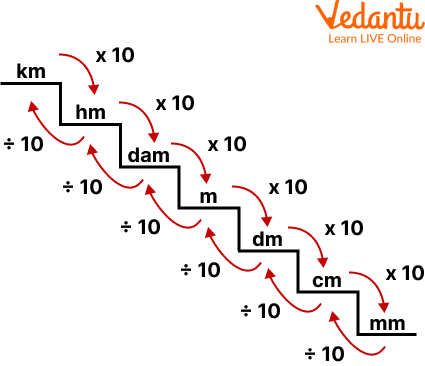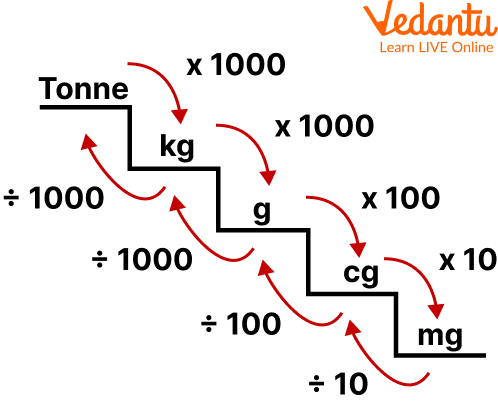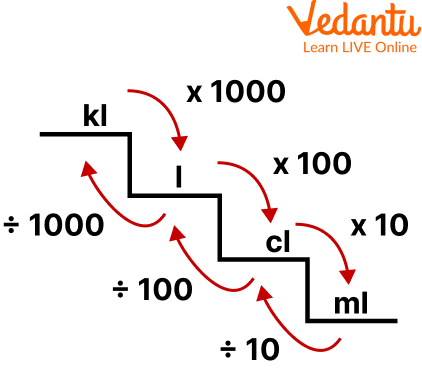Courses
Courses for Kids
Free study material
Offline Centres
More

# Metric Conversion ChartLast updated date: 30th Nov 2023
Total views: 97.5k
Views today: 2.97k## An Overview of Metric System Chart

The most common method for determining height, distance, and other daily items is the metric system of measurement. Let's use a jar of milk as an example. To determine the amount of milk, we use litres, and to determine the height of the jar, we use metres (or centimetres). This is because these metric units, also known as SI units, are widely used throughout the world (International System of Units). Come on, let's start learning about the metric system. The man who is credited with creating the metric system of measurement is Gabriel Mouton. English clergyman John Wilkins proposed a system for measuring length, mass, area, and volume in 1668.

## Metric Unit

For measuring length, mass, area, and capacity, different metric units are used. For instance, the metric units used to measure length are millimetres, centimetres, metres, and kilometres. The weight is measured in grams and kilograms. View the metric table to learn about all the metric system units used for various applications.

For measurement of Length

 S.No. Metric Units Common Abbreviation 1. Millimetres mm 2. Centimetres cm 3. Metres m 4. Kilometres km

For measurement of Capacity or litre chart

 S.No. Metric Units Common Abbreviation 1. Milliliters ml 2. Centiliters cl 3. Litres l 4. Kiloliters kl

For measurement of Mass or volume conversion

 S.No. Metric Units Common Abbreviation 1. Milligrams mg 2. Centigrams cg 3. Grams g 4. Kilograms kg 5. Tonne t

For measurement of Area

 S.No. Metric Units Common Abbreviation 1. Square centimetres sq.cm 2. Square metres sq.m 3. Square kilometres sq.km 4. Hectare ha

## Metric System Chart

The formulas for converting between different metric units are contained in the metric system chart. By exploring its multiplying factor, you can quickly convert one unit to another. For example: you can see from the metric system chart that 1 metre equals 100 centimetres. By multiplying 5 metres by 100, you can convert 5 metres to centimetres using this formula.

For length:Conversion chart for length

1. 1 cm = 10 mm

2. 1 mm = 0.1 cm

3. 1 m = 100 cm

4. 1 cm = 0.01 m

5. 1 km = 1000 m

6. 1 m = 0.001 km

For Mass:Conversion chart for mass

1. 1 cg = 10 mg

2. 1 mg = 0.1 cg

3. 1 g = 100 cg

4. 1 cg = 0.01 g

5. 1 g = 1000 mg

6. 1 mg = 0.001 g

7. 1 kg = 1000 g

8. 1 g = 0.001 kg

9. 1 tonne = 1000 kg

10. 1 kg = 0.001 tonne

For Capacity:Conversion chart for capacity

1. 1 cl = 10 ml

2. 1 ml = 0.1 cl

3. 1 l = 100 cl

4. 1 cl = 0.01 l

5. 1 l = 1000 ml

6. 1 ml = 0.001 l

7. 1 kl = 1000 l

8. 1 l = 0.001 kl

## Solved Examples

Q 1. Convert 2000 l into kl?

Ans: We are given a measurement in the form of litre i.e. $2000l$. As we know that

$1000 l=1 kl$. So we will divide the expression by 1000. So that we will convert them into expressions.

Step2: By simply following the unitary method, we will find the value:

If $1000 l=1 kl$

Then $1 l=\dfrac{1}{1000} kl$

Hence, the value of $2000 l=\dfrac{1 \times 2000}{1000}$

$=2 kl$

Hence the value is $2 kl$

Q 2. Convert 500 metres to kilometres.

Ans: Both metres and kilometres are metric system units of measuring distance. In $1 \mathrm{~m}$, there is $\dfrac{1}{1000} \mathrm{~km}$.

$\Rightarrow 1 \mathrm{~m}=\dfrac{1}{1000} \mathrm{~km}$

$\Rightarrow 500 \mathrm{~m}=\dfrac{500}{1000} \mathrm{~km}$

$\Rightarrow 500 \mathrm{~m}=\dfrac{1}{2} \mathrm{~km}=0.5 \mathrm{~km}$

Therefore, 500 metres equals half a kilometre or $0.5 \mathrm{~km}$.

Q 3: How many centigrams are there in 12 grams?

Ans: Centigrams and grams are the units of the metric system. In 1 gram, there are 100 centigrams.

$\Rightarrow 1 \mathrm{~g}=100 \mathrm{cg}$

$\Rightarrow 12 \mathrm{~g}=12 \times 100 \mathrm{cg}$

$\Rightarrow 12 \mathrm{~g}=1200 \mathrm{cg}$

Therefore, there are 1200 centigrams in 12 grams.

## Practice Problems

Q 1. Convert 55 ml into l. (Ans: 0.055 l)

Q 2. Convert 21 kg 46 g into kilograms. (Ans: 21.046 kg)

## Summary

As discussed above, The metric system of measurement is the most popular way to determine the height, distance, and other everyday items. The common measurement system that uses decimal values is the metric system. It uses powers of ten for unit conversions. The metric system of units can be used to measure length, weight, area, capacity, speed, distance, time, etc. In the metric system, length is measured in metres, centimetres, and kilometres; weight is measured in kilogrammes, milligrammes, and grammes; capacity is measured in litres, kiloliters, centilitres, and millilitres; and time is measured in hours, minutes, and seconds.

## FAQs on Metric Conversion Chart

1. What Distinguishes Imperial and Metric Units?

The metric system uses both metres and grammes as units of measurement. The imperial system measures things in feet, inches, and pounds. The imperial system, which uses measurements in feet, inches, and pounds, is used in the United States, as opposed to most other nations, which use the metric system, which uses measuring units like metres and grammes. The metric system uses powers of 10. For instance, 100 cm equals 1 m, 1000 g equals 1 kg, etc. The imperial units don't follow any special pattern.

2. What are the Conversion Factors?

The approach of converting something from one form to another in mathematics, such as from inches to millimetres or from litres to gallons, is known as conversion. The following measurements: length, weight, capacity, temperature, and speed are all measured in units. A conversion factor is a number used to multiply or divide one set of units into another. If a conversion is required, it must be done using the correct conversion factor to get an equal value. For instance, 12 inches equals one foot when converting between inches and feet.

3. How Are Metric Conversion Tables Memorized?

The metre (m), kilogramme (kg), second (s), kelvin (K), ampere (A), mole (mol), and candela are the seven fundamental units of measurement in the SI system (cd). The fundamentals can be remembered as Deci, which is 10 times smaller than Centi, 100 times smaller than Milli, and 1,000 times smaller than metres, litres, and kilograms in the SI system. King Henry Doesn't Usually Drink Chocolate Milk is a useful mnemonic for remembering the crucial metric prefixes.